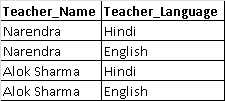# BCNF, 4NF and 5NF

First three normal forms are important from exam point of view; we will discuss other normal forms viz. BCNF, 4NF and 5NF for sake of completeness. If you are not familiar about concept of normalization, do go through them first:

Boyce-Codd normal form (BCNF) named after its creators is based on the concept of determinant.

#### What is determinant?

We know that if B is functionally dependent on A, that is, A functionally determines B then in such case A is called determinant.

#### BCNF

A table is in BCNF form if the only determinants in the table are the candidate keys. It means every column, on which some other column is fully functionally dependent, is also a candidate for the primary key of the table.

#### Fourth Normal Form

A table is in the fourth normal form (4NF) if:

• It is in BCNF.
• It does not have any independent multi-valued parts of the primary key.

Let’s say we have table Teacher which gives information about:

1. A teacher can teach many teachers.
2. A teacher may know many languages.

We can see that Narendra is teaching three subjects and knows two languages, thus there are two independent multi-valued dependencies. We can split the table into two tables.

Table: TeacherSubjectTable: TeacherLanguageYou can see know that there is less data redundancy.

#### Fifth Normal Form (5NF)

A table is in the fifth normal form (5NF), if:

• It is in the fourth normal form.
• Every join dependency in the table is implied by the candidate keys.

This normal form is theoretical in nature, so we will not discuss it further. That concluded the discussion of normalization.